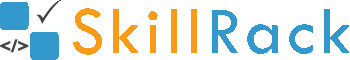Must Solve 100 Programs for Campus Placement Part - 2/104 months ago

1) Two Numbers Equal

The program must accept two numbers and print yes if they are equal. Else the program must print no as the output.

Example Input/Output 1:
Input:
40 40

Output:
yes

Example Input/Output 2:
Input:
50 90

Output:
no

Click the link below to solve this program
http://www.skillrack.com/solve/7876

2) Largest of Two Numbers

The program must accept two numbers and print the largest number.

Example Input/Output 1:
Input:
12 25

Output:
25

Example Input/Output 2:
Input:
9 5

Output:
9

Click the link below to solve this program
http://www.skillrack.com/solve/7877

3) Print Remainder when Divided by 5

The program must accept a number and print its remainder when it is divided by 5.

Example Input/Output 1:
Input:
12

Output:
2

Example Input/Output 2:
Input:
10

Output:
0

Click the link below to solve this program
http://www.skillrack.com/solve/7878

4) Pass or Fail

The program must accept a mark and print if it is pass or fail. The pass mark is 40.

Example Input/Output 1:
Input:
40

Output:
pass

Example Input/Output 2:
Input:
32

Output:
fail

Click the link below to solve this program
http://www.skillrack.com/solve/7875

5) Multiply Two Numbers

Accept two numbers and print their product.

Example Input/Output 1:
Input:
4 5

Output:
20

Example Input/Output 2:
Input:
11 12

Output:
132

Click the link below to solve this program
http://www.skillrack.com/solve/7879

6) Multiply Three Numbers

Accept three numbers and print their product.

Example Input/Output 1:
Input:
2 5 10

Output:
100

Example Input/Output 2:
Input:
6 3 7

Output:
126

Click the link below to solve this program
http://www.skillrack.com/solve/7883

7) Print Unit Digit

The program must accept a number and print its unit digit.

Example Input/Output 1:
Input:
72

Output:
2

Example Input/Output 2:
Input:
65

Output:
5

Click the link below to solve this program
http://www.skillrack.com/solve/7881

8) Smallest of Two Numbers

The program must accept two numbers and print the smallest number.

Example Input/Output 1:
Input:
14 23

Output:
14

Example Input/Output 2:
Input:
5 1

Output:
1

Click the link below to solve this program
http://www.skillrack.com/solve/7882

9) Character - Male or Female

The program must accept a character.
- If the character is m, print male
- If the character is f, print female

Click the link below to solve this program
http://www.skillrack.com/solve/7884

10) Remove Unit Digit

The program must accept a number and remove the unit digit.

Note: The number is greater than 9.

Example Input/Output 1:
Input:
102

Output:
10

Example Input/Output 2:
Input:
12345

Output:
1234

Click the link below to solve this program
http://www.skillrack.com/solve/7880